# The truth table for the following logic circuit is:Question:

The truth table for the following logic circuit is: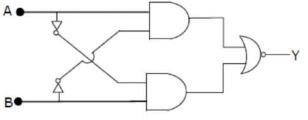1. (1)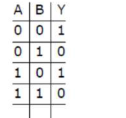2. (2)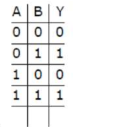3. (3)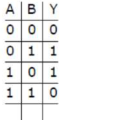4. (4)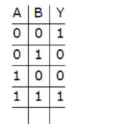Correct Option: , 4

Solution:

(4)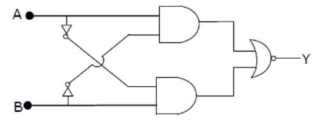If $A=B=0$ then output $y=1$

If $A=B=1$ then output $y=1$